output.to from Sideway
Draft for Information Only

# Content

```  MatLab: Scratch Canvas & Graphic Operations   2D Plots   Line Graphs    Examples       3D Plots```

## MatLab: Scratch Canvas & Graphic Operations

Major Reference Source: MatLab Verson 7.0

Using MatLab package as a scratch canvas interactively is one of the common application of the MatLab package. MatLab commands are entered at the prompt command, >>, of the Command Window through the PC keyboard. Both entered commands and evaluation results are displayed in the Command Window. All new line or lines of commands or instructions after the prompt command will be passed to MatLab for evaluation after pressing the Enter key no matter the position of mouse cursor is at the end of line or lines or not. However, the MatLab package is design as a tool to manipulate data instead of a design tool to create some common features, therefore functions are usually some basic tools to present or display the provided data graphically in the scratch canvas as a figure. In other words, both the domain and range of the function are needed to be provided before a single graph of the function can be presented by a plotting function. As the plotting area is only be considered as a scratch canvas, multiple functions plotting on the same scratch canvas are allowed. Besides there are also addition tools for annotating and manipulating the figure in the scratch canvas.

### 2D Plots

There are five types of 2D presentation, namely, Line Graph, Bar Graph, Area Graph, Direction Graph and Scatter Graph. These 2D presentations are characteristic by its form of presentation.

### Line Graphs

The presentation of plot is in the form of line, actually piecewise line. The matrix and array arithmetic includes seven types of operations:

Function Description
plot(x,y) to present one group of 2D data set, y with respect to x,  by joining pointwise data with line in linear scales for both axes.
plotxy(x1,y1,x2,y2) to present Two different groups of 2D data sets, y with respect to x, by joining pointwise data with line based on two different y-axes and in linear scales for both axes.
loglog(x,y) to present one group of 2D data set, y with respect to x, by joining pointwise data with line in logarithmic scales for both axes.
semilogy(x,y) to present one group of 2D data set, y with respect to x, by joining pointwise data with line in linear scale for x axis and in logarithmic scale for y axis.
semilogx(x,y) to present one group of 2D data set, y with respect to x, by joining pointwise data with line in logarithmic scale for x axis and in linear scale for y axis.
stairs(x,y) to present one group of 2D data set, y with respect to x, by drawing a stair step starting from horizontal line between two consecutive pointwise data in linear scales for both axes.
contour(z) to present one group of 3D data set, z with respect to x and y, by drawing contour lines according to the third dimension, data value z of pointwise data automatically with scale axes respect to the first two dimension, x and y index of pointwise data in linear scales for both axes.
ezplot(f1,f2) to present the line plotting of a function expression within an automatically generated default domain. The function expression with f1 and f2 is used to represent two parametrically defined planar function fx(t) and fy(t) over thte default domain 0<t<2π. The function expression with f1 only is used to represent either an explicit function with respect to x over the default domain -π<x<2π or an implicit function of x and y over the default domain -π<x<2π and -π<y<2π.
ezcontour(f) to present the contour lines plotting of a function expression within an automatically generated default domain. The function expression with f1 and f2 is used to represent two parametrically defined planar function fx(t) and fy(t) over thte default domain 0<t<2π. The function expression with f1 only is used to represent either an explicit function with respect to x over the default domain -π<x<2π or an implicit function of x and y over the default domain -π<x<2π and -π<y<2π.

#### Examples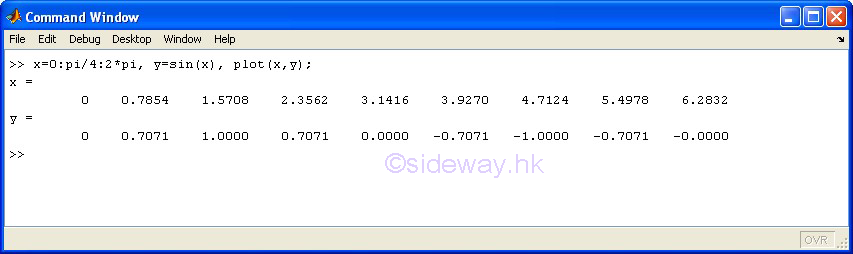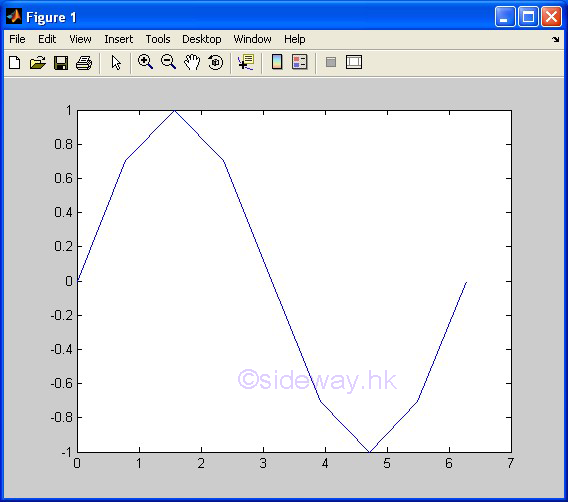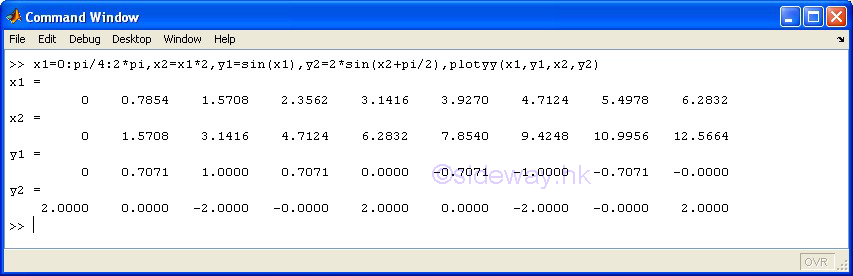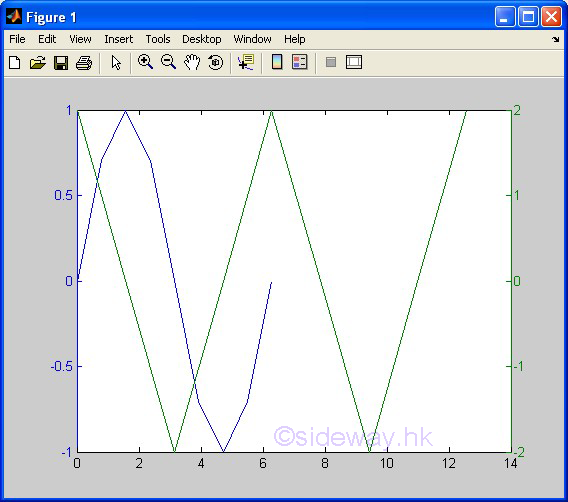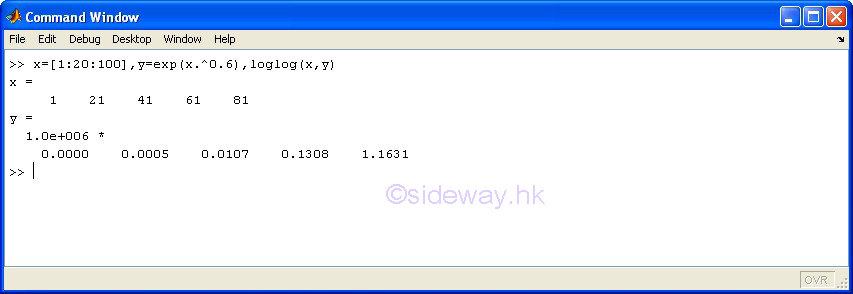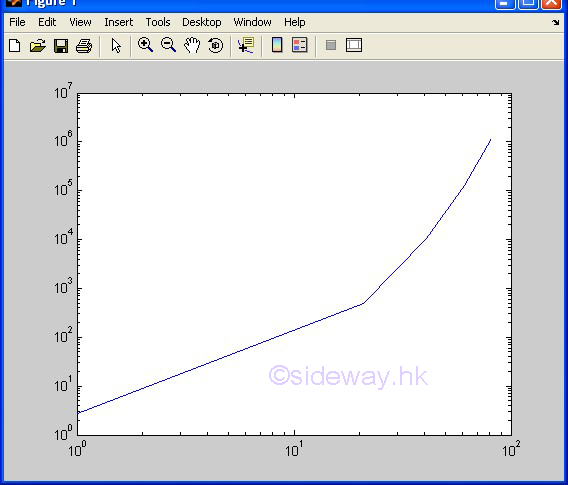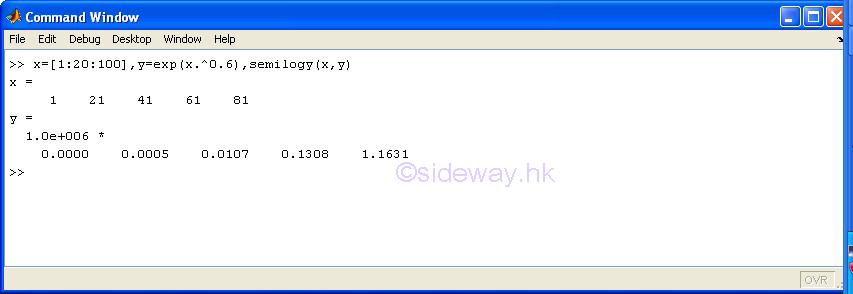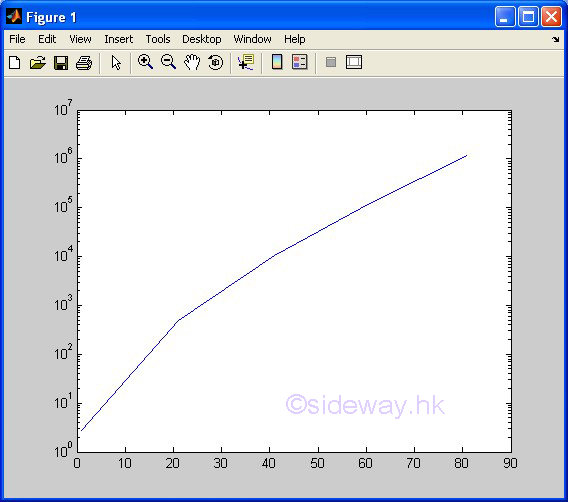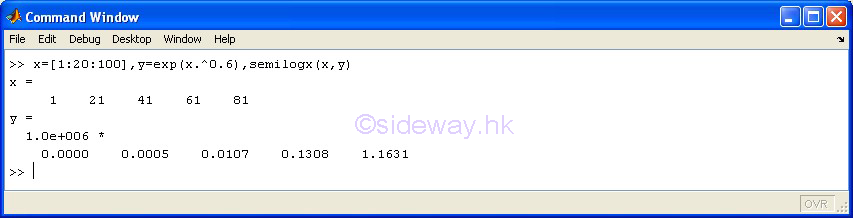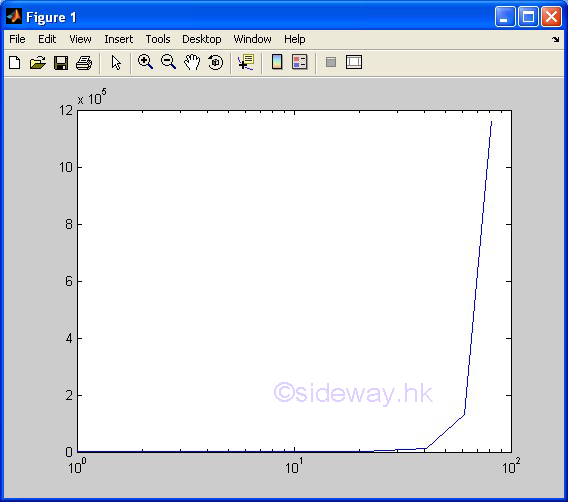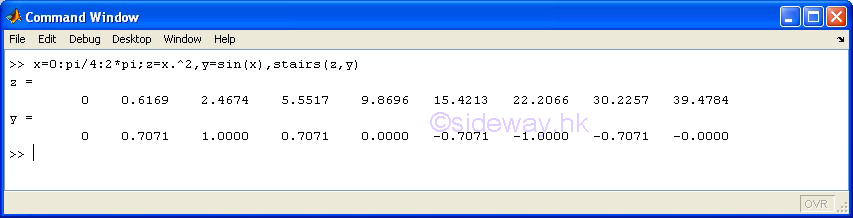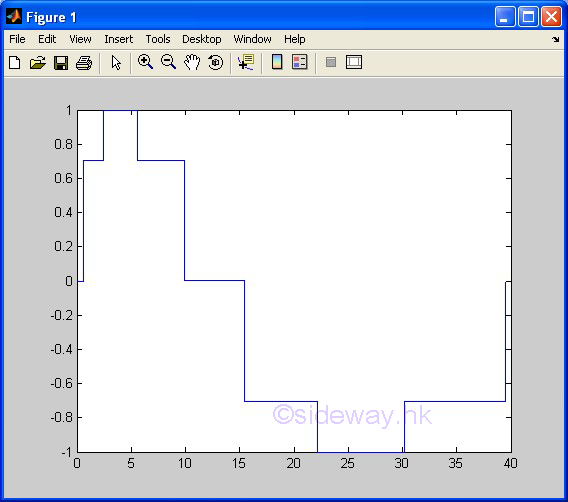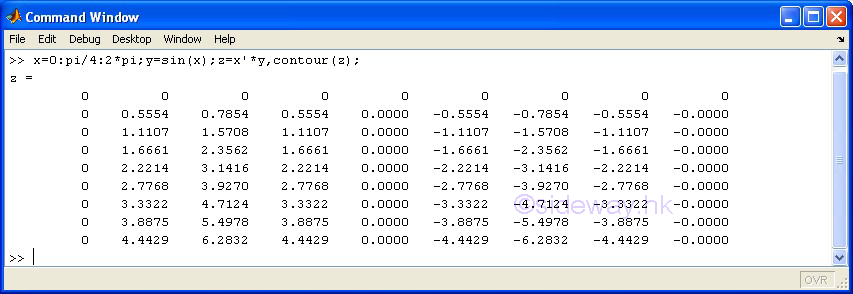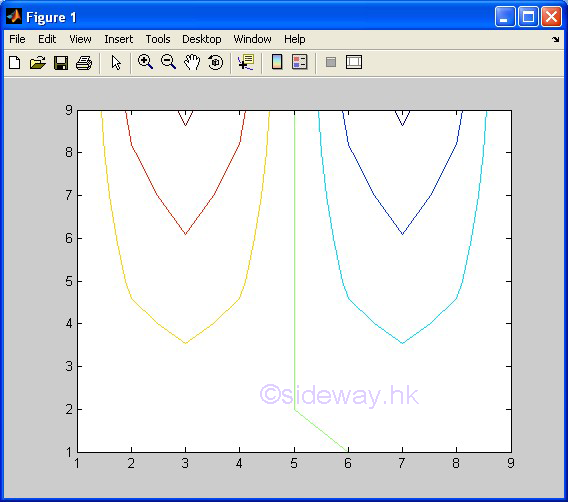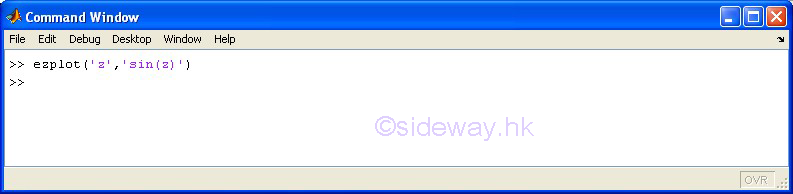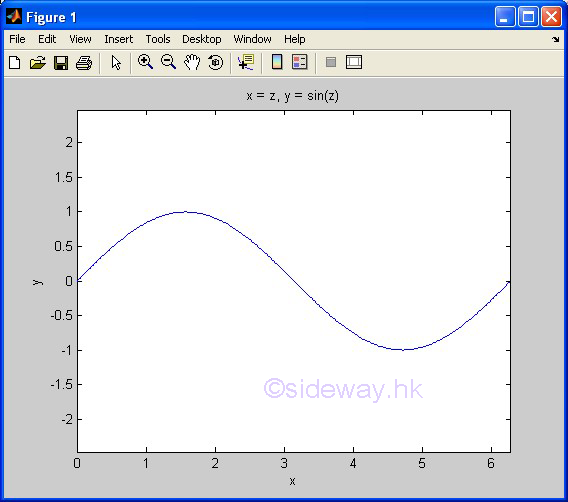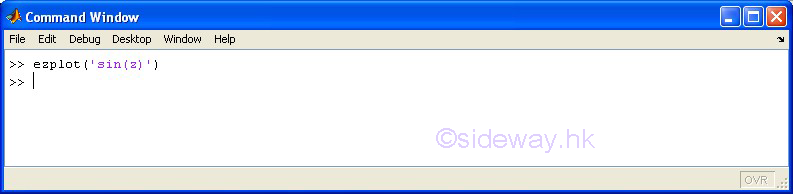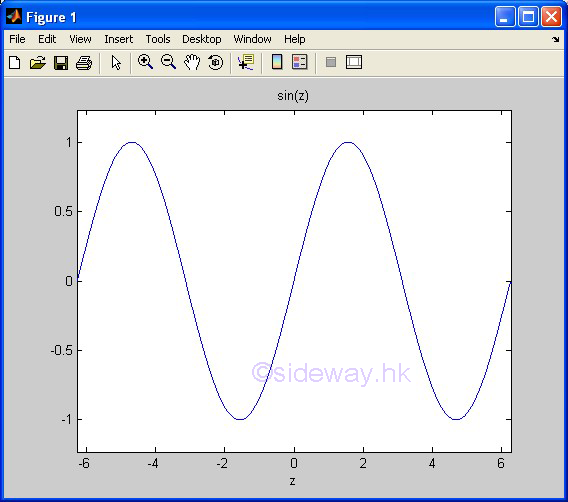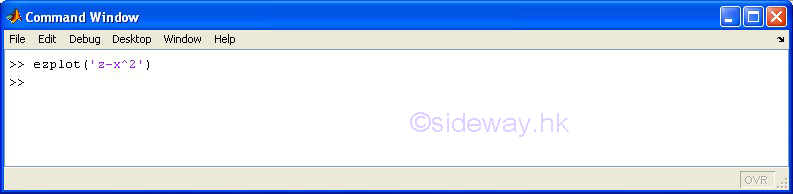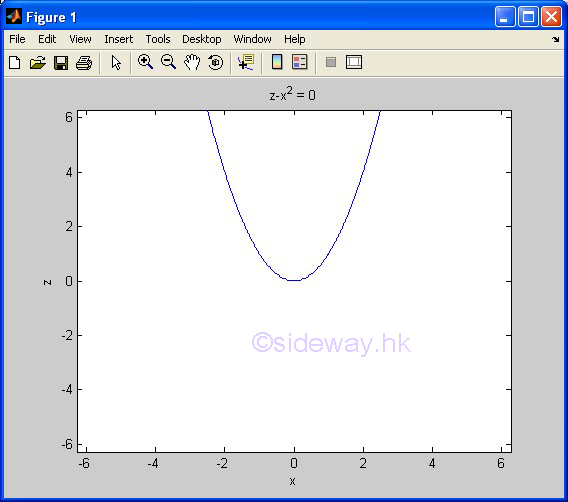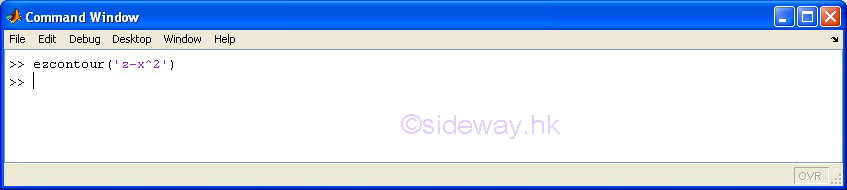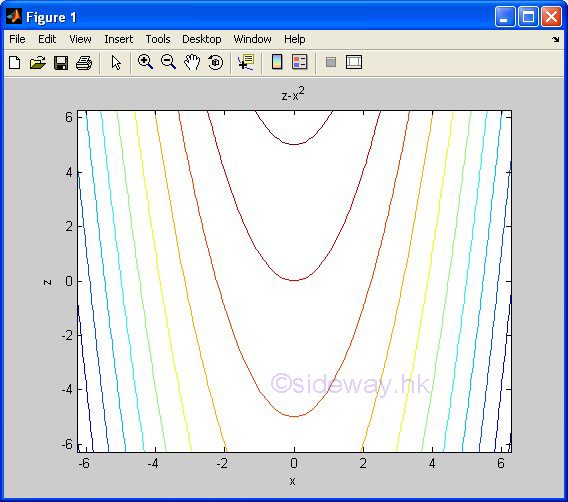### 3D Plots

ID: 140600009 Last Updated: 6/19/2014 Revision: 0Home 5

Management

HBR 3

Information

Recreation

Culture

Chinese 1097

English 339

Computer

Hardware 249

Software

Application 213

Latex 52

Manim 205

KB 1

Numeric 19

Programming

Web 289

Unicode 504

HTML 66

CSS 65

SVG 46

ASP.NET 270

OS 429

Python 72

Knowledge

Mathematics

Algebra 84

Geometry 34

Calculus 67

Engineering

Mechanical

Rigid Bodies

Statics 92

Dynamics 37

Control

Natural Sciences

Electric 27